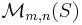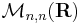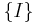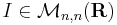# Leontief input–output model

The Leontief input–output model is a long-run economic growth model.

## Variables in the model

Below,$\mathcal M_{m,n}(S)$ denotes an m by n (m rows and n columns) matrix with elements taken from the set S.

Name Variable Unit Set of possible values Rival input? Variable type Notes
Number of sectors n$\mathbf N$
A$\mathcal M_{n,n}(\mathbf R)$
d$\mathcal M_{n,1}(\mathbf R)$
x$\mathcal M_{n,1}(\mathbf R)$
I$\{I\}$ where$I \in \mathcal M_{n,n}(\mathbf R)$

## Mathematical formalism$x = Ax + d$